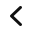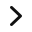# How to Teach the Area of Irregular Shapes

My class has been learning to find the area of shapes for a few weeks now.  But now it was time to move on to find the area of irregular shapes.  So far, my students have learned how to count tiles or squares or to multiply length times width.  But I’m upping the ante to tackle this particular common core standard:

Common Core Standard 3.MD.C.7.d
Recognize area as additive.  Find areas of rectilinear figures by decomposing them into non-overlapping rectangles and adding the areas of the non-overlapping parts, applying this technique to solve real-world problems.

## What does that all mean?

Hmmm….ok..but what exactly does that mean?  It means that students should use the Distributive Property of Multiplication to bisect irregular shapes into rectangles. So to find the area of irregular shapes you first find the area of each rectangle. Then you add those areas together to find the total area of the irregular shape.

But does it mean that if students are given an NON-tiled shape (meaning no tiles to count, but labeled side lengths and widths with measurements), they are to also find missing or non-labeled lengths?

Good question.  I tried that and it was way TOO HARD for my students.  Even the other third-grade team members said that it was also too hard to do for their students.

## How Then, Did I Teach Finding the Area of Irregular Shapes?

Let’s look at the progression of my lessons to find the area of irregular shapes. It was a two-day lesson on which the first day was just practicing and reviewing the Distributive Property of Multiplication to find the area of REGULAR shapes.

Day two, focused on finding the area of irregular shapes.  I gave each student an irregular shape, a sticky note for doing calculations and showing work and the instructions were simple:  Find the area of the irregular shape.

After the students had cut out the irregular shape, I heard nothing but crickets for a few minutes.  So I started giving them some guidance.  You can do anything with the shape: fold it, color it, cut it.  That turned on a few light bulbs.

One student decided she could fold it.  Great start!

## Cutting Apart to Find the Area of Irregular Shapes

Many students decided to cut it apart.  They were not all cut in the same way, but all did end up with two regular rectangles.

“So what are you going to do next?” I asked.  I told them that this should look familiar from the previous day’s lesson.  More light bulbs went off.

## Can You Explain How You Got This Answer?

One student came up with this on the sticky note.  My next question to him:  Where did these numbers come from?  How do you know that answer is correct?

Another student did something similar but actually showed multiplication.   I told the student that he was on the right track!

Here’s a little trick I like to use with my class when I see that several students are on the right path.  I take a picture of their work with my iPhone.  Then I use the AirPlay feature of my iPhone to send it directly to my projector so the rest of the students can see what was done.

I also have the students explain their thinking.  This is also a good place to use the Math Talk techniques and have students repeat what a student said, or add to what a student said or disagree with what a student said.

## But How Did You Find the Total Area of an Irregular Shape?

At this point, my guiding question was this.  You’ve cut apart the irregular shape.  You know how to find the area of each rectangle because it’s just an array and so you can use multiplication.  But how do you get the total area of the irregular shape?  The discussion eventually went to the idea that we are joining the two areas back together, which in math is called addition!

From there, it was just a perfect segue into the writing a Distributive Property of Multiplication sentence.

So let’s review what we just did.

## And Now, We Hit a Speed Bump!

The lesson continued with finding the area of irregular shapes without the tiles.  This is where things just started to get too difficult for my students.  In my teaching plan, I assumed since they knew how to find a missing side length when we studied perimeter, that they knew how to use the Distributive Property of Multiplication and that they knew how to find the area.

So I figured removing the tiled squares would be the next step.  Boy, was I wrong!

Above is the example I used.  I had them also take out their math journals and copy the shape into their math journals and label it.

## I Modeled How to Find the Area of Irregular Shapes for Their Math Journals

Then, I proceeded to work with them by demonstrating how to bisect the irregular shape into two regular rectangles.  Next, I show them how to figure out how to label the sides that are not labeled by using subtraction and reasoning.  Finally, using the formula of length times width, find each area and then add them using the Distributive Property of Multiplication.  So simple, right! Nope!

I told my students to keep this math journal example out on their desks as we went to a worksheet to start some guided practice.  But even with the guided practice, it was too hard!

They really struggled with finding the correct sides to multiply or reasoning to find the length of an unlabeled side.

## Back to the Familiar!

I could tell that just after two problems, there are just too many steps involved in solving this type of problem.  So at this point, I abandoned this worksheet and went back to the math book and had the students continue practicing with tiled shapes using the Distributive Property of Multiplication.

The students will practice with shapes that are not tiled.  But I will label EACH length and width to lessen the steps involved.   It is that step, figuring out missing sides of irregular shapes that really confuses the students.

## Don’t Go Yet!

Here’s another blog post on area and perimeter that might interest you.

Are you looking for resources for teaching area and perimeter? Check out these resources from my TpT Store!Area and Perimeter Resources
Area and Perimeter Resources
Area and Perimeter Resources
Area and Perimeter Resources
Area and Perimeter Resources
Area and Perimeter Resources
Area and Perimeter Resources
Area and Perimeter Resources
Area and Perimeter Resources
Area and Perimeter ResourcesDo your students confuse area and perimeter?

I created this poster (color and greyscale) to help students make sense of what’s area and what’s perimeter. It comes with 10 suggestions for use.